### Home > PC3 > Chapter 8 > Lesson 8.3.4 > Problem8-138

8-138.
1. Rewrite each expression as the sine, cosine, or tangent of an angle. Homework Help ✎

1.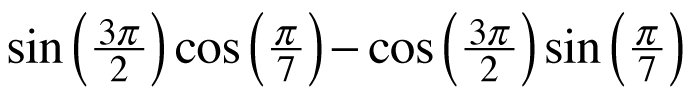2.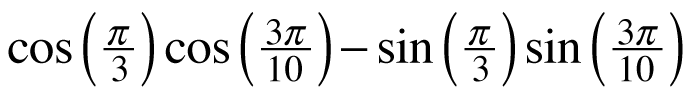3.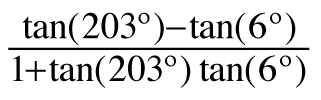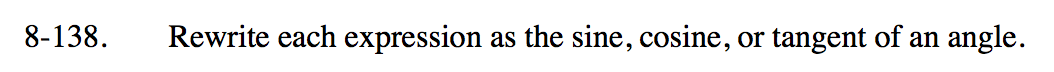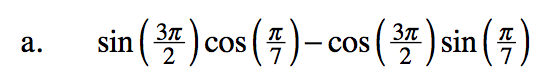Look at the angle difference formula for sine.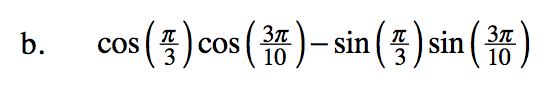Look at the angle sum formula for cosine.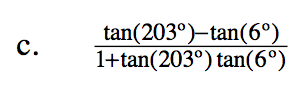Look at the angle difference formula for tangent.